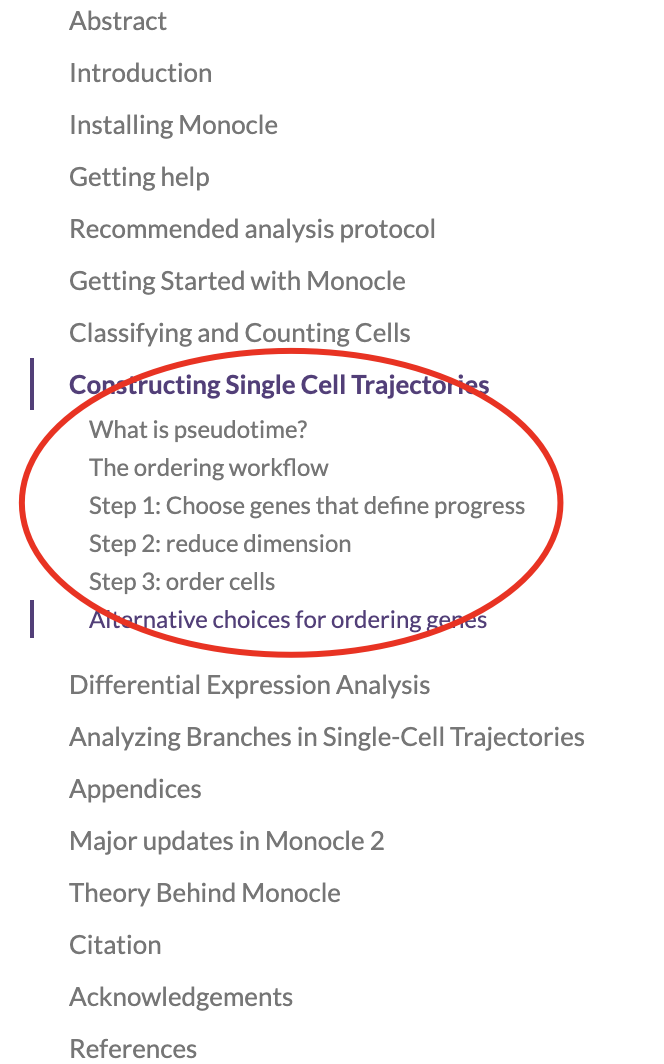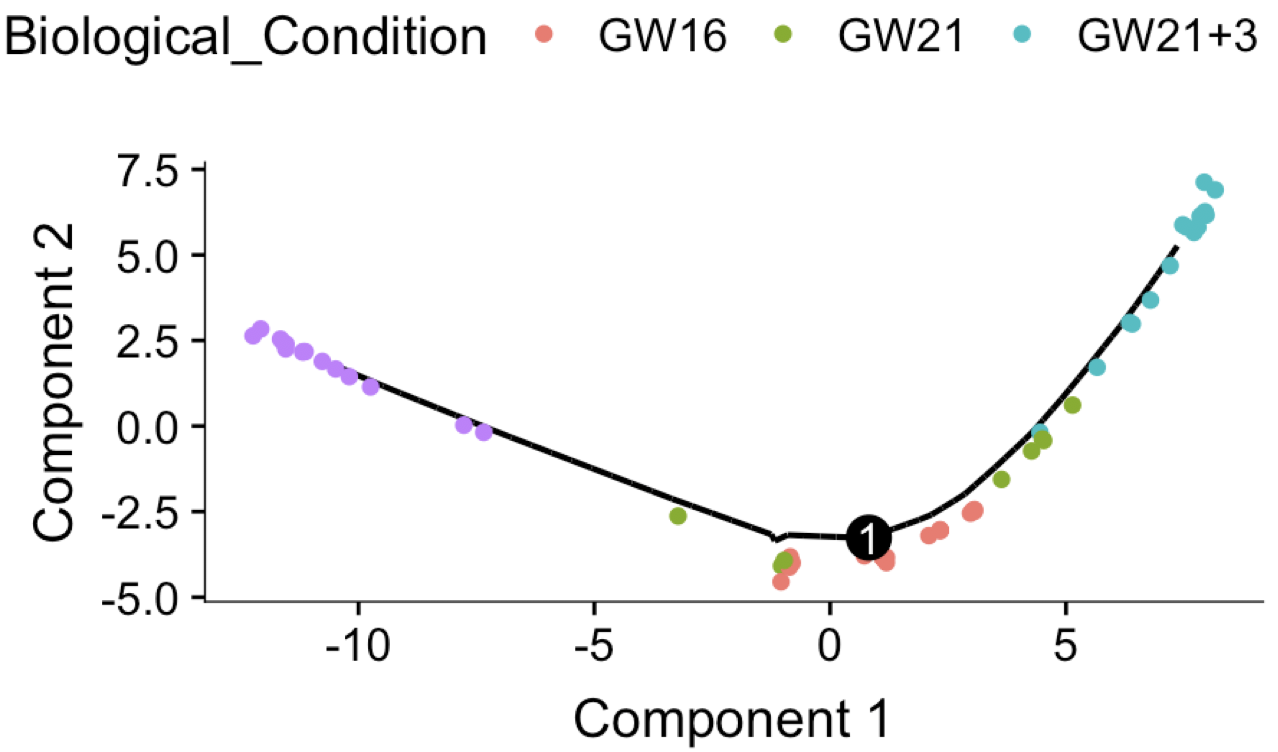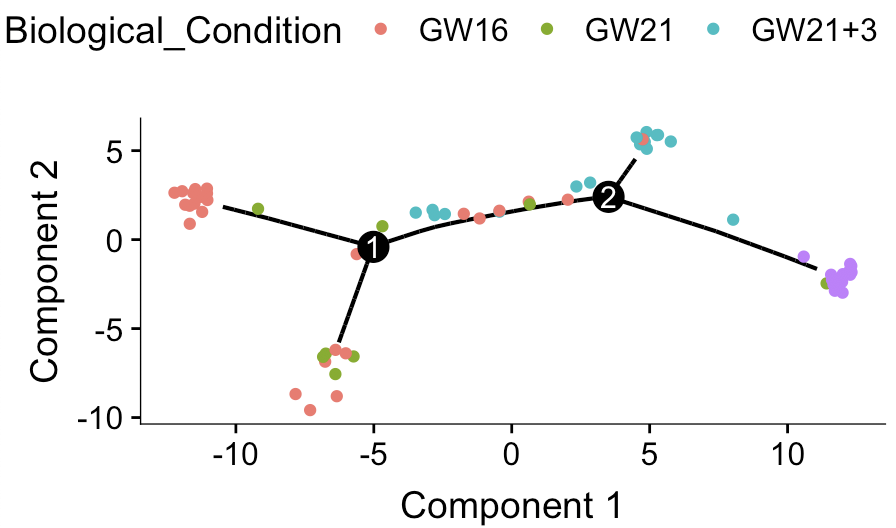# 使用monocle做单细胞谱系发育

• step1: 创建对象

• step2: 质量控制

• step3: 表达量的标准化和归一化

• step4: 去除干扰因素(多个样本整合)

• step5: 判断重要的基因

• step6: 多种降维算法

• step7: 可视化降维结果

• step8: 多种聚类算法

• step9: 聚类后找每个细胞亚群的标志基因进行亚群命名

• step10: 继续分类### 同样我们首先拿scRNAseq包的表达矩阵测试

#### 首先拿到表达矩阵和表型信息

``````library(monocle)
library(scRNAseq)
## ----- Load Example Data -----
data(fluidigm)
ct <- floor(assays(fluidigm)\$rsem_counts)
ct[1:4,1:4]
sample_ann <- as.data.frame(colData(fluidigm))
table(sample_ann\$Coverage_Type)
table(sample_ann\$Biological_Condition)
kp=sample_ann\$Coverage_Type=='High'
ct=ct[,kp]
sample_ann=sample_ann[kp,]
``````

#### 然后构建monocle需要的对象

``````gene_ann <- data.frame(
gene_short_name = row.names(ct),
row.names = row.names(ct)
)

pd <- new("AnnotatedDataFrame",
data=sample_ann)
fd <- new("AnnotatedDataFrame",
data=gene_ann)

sc_cds <- newCellDataSet(
ct,
phenoData = pd,
featureData =fd,
expressionFamily = negbinomial.size(),
lowerDetectionLimit=1)
sc_cds
``````

``````sc_cds <- estimateSizeFactors(sc_cds)
sc_cds <- estimateDispersions(sc_cds)
``````

#### 轨迹分析需要指定基因

``````if(F){
disp_table <- dispersionTable(cds)
unsup_clustering_genes <- subset(disp_table,
mean_expression >= 0.1)
cds <- setOrderingFilter(cds, unsup_clustering_genes\$gene_id)
dim(cds)
diff_test_res <- differentialGeneTest(cds,
fullModelFormulaStr = "~Biological_Condition")
# 哪怕仅仅是65个单细胞，monocle的这个differentialGeneTest函数运行也不快。
ordering_genes <- row.names (subset(diff_test_res, qval < 0.01))
save(ordering_genes,file = 'ordering_genes_by_Biological_Condition_high.Rdata')
}
``````

#### 使用官网代码构建谱系发育

``````cds <- setOrderingFilter(cds, ordering_genes)
plot_ordering_genes(cds)
# 然后降维
cds <- reduceDimension(cds, max_components = 2,
method = 'DDRTree')
# 降维是为了更好的展示数据。
# 降维有很多种方法, 不同方法的最后展示的图都不太一样, 其中“DDRTree”是Monocle2使用的默认方法
# 接着对细胞进行排序
cds <- orderCells(cds)
## 最后两个可视化函数
plot_cell_trajectory(cds, color_by = "Biological_Condition")
# 可以很明显看到细胞的发育轨迹
# 还有几个其它可视化函数，我们明天介绍
plot_cell_trajectory(cds, color_by = "State")
plot_cell_trajectory(cds, color_by = "Pseudotime")
plot_cell_trajectory(cds, color_by = "State") +
facet_wrap(~State, nrow = 1)
``````### 选择错误的基因集去做轨迹分析会怎么样呢？

``````# 加入为了方便起见，直接挑选top2000的MAD基因。
``````